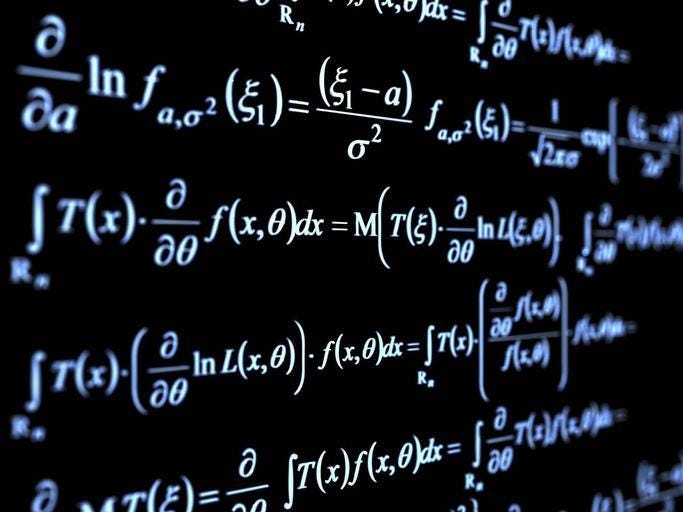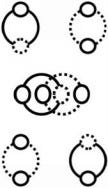# The Mathematical Genius

X and Y are diameter and circumference, zero and one, etc.A string of characters articulates a line (diameter of a circle).

X and Y are diameter and circumference, literally and figuratively (zero and one).

Therefore, one is two (zero is one) (circumference is diameter).One and Two (Circumference and Diameter) (Two Personalities, All of Us)

This is the basis for everything (perpetual motion is absolute zero) (X and Y is X and X) (X and X’) (duplication) (negation). The constant is a variable (the variable is constant) (circularity is ubiquitous).